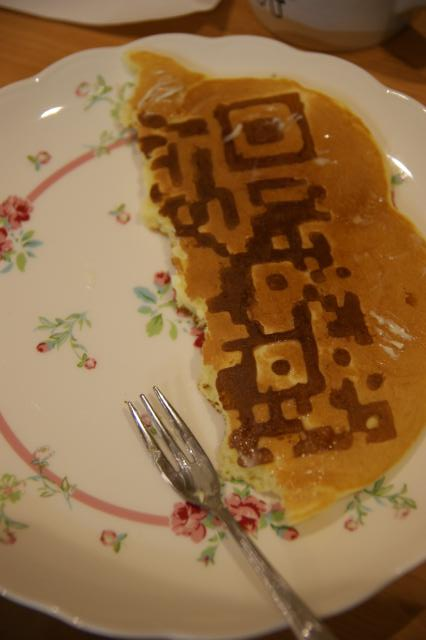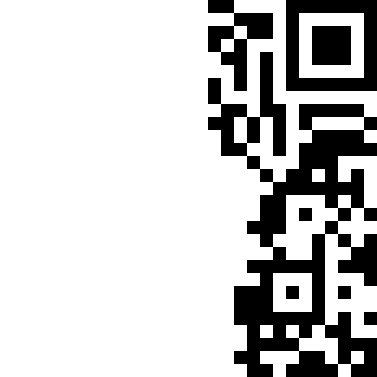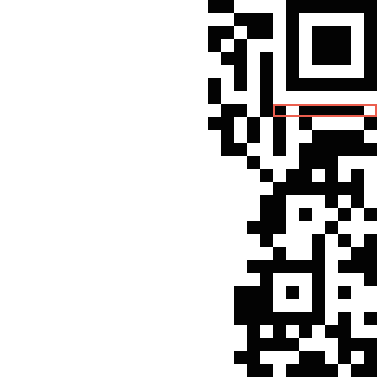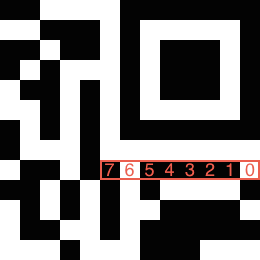## QR200 - QR (Easy)

Funniest joke in the world(?):
“Last night, I had a dream I was eating QR cakes….
but when I woke up, half my QR code was gone!”## First Look

At first, we need clean image based on the image above.The size of this QR code is 29x29 and the size of version V is N × N with N = 17 + 4V, so this is version 3.

## Format InformationNote that the area indicates format information of QR code. Actually the format information is 15 bits long and the area has last 8 bits.Searching the list of all format information strings, we can find out that the type information bits are `001001110111110`. So this QR code has ECC level H and mask pattern 1.

According to QR Mask Patterns Explained, mask number 1 has formula `(row) mod 2 == 0`. Note that the row number start from 0. So we have to switch the bit of the row of which the coordinate is 0, 2, 4, …, 28.

Also there are fixed patterns in QR code, so we have to switch bits of data section only. See the data area and the bit order.(via wikipedia.org)

So, the raw D1–D26 are:

``````D1  = 0b11101100
D14 = 0b10000010
D2  = 0b11111000
D15 = 0b10010101
D3  = 0b00110110
D16 = 0b00111101
D4  = 0b01110110
D17 = 0b01100010
D5  = 0b00100010
D18 = 0b11101001
D6  = 0b11110001
D19 = 0b10100001
D7  = 0b00110111
D20 = 0b11100101
D8  = 0b01010010
D21 = 0b11010101
D9  = 0b00010111
D22 = 0b00101101
D10 = 0b11011110
D23 = 0b10010111
D11 = 0b01000100
D24 = 0b10001011
D12 = 0b01010100
D25 = 0b01111000
D13 = 0b11001101
D26 = 0b11000110
``````

``````D1  = 0b00100000
D14 = 0b01001110
D2  = 0b00110100
D15 = 0b01011001
D3  = 0b11111010
D16 = 0b00001110
D4  = 0b01000101
D17 = 0b01010001
D5  = 0b00010001
D18 = 0b11011010
D6  = 0b00111101
D19 = 0b10010010
D7  = 0b00000100
D20 = 0b11010101
D8  = 0b10011110
D21 = 0b00011001
D9  = 0b11010100
D22 = 0b00010001
D10 = 0b00010100
D23 = 0b00001110
D11 = 0b11011101
D24 = 0b00010010
D12 = 0b11010010
D25 = 0b00011111
D13 = 0b01010100
D26 = 0b01000000
``````

Now we can start decoding the data.

## Data Decoding

There are mode indicators for decoding:

• `0001`: Numeric Mode (10 bits per 3 digits)
• `0010`: Alphanumeric Mode (11 bits per 2 characters)
• `0100`: Byte Mode (8 bits per character)
• `1000`: Kanji Mode (13 bits per character)
• `0111`: ECI Mode

Character count indicator follows after a mode indicator.

• Version 1–9
• Numeric mode: 10 bits
• Alphanumeric mode: 9 bits
• Byte mode: 8 bits
• Kanji mode: 8 bits
• Version 10–26
• Numeric mode: 12 bits
• Alphanumeric mode: 11 bits
• Byte mode: 16 bits
• Kanji mode: 10 bits
• Version 27–40
• Numeric mode: 14 bits
• Alphanumeric mode: 13 bits
• Byte mode: 16 bits
• Kanji mode: 12 bits

See the encoding process for each mode:

``````data = '00100000' \
'00110100' \
'11111010' \
'01000101' \
'00010001' \
'00111101' \
'00000100' \
'10011110' \
'11010100' \
'00010100' \
'11011101' \
'11010010' \
'01010100' \
'01001110' \
'01011001' \
'00001110' \
'01010001' \
'11011010' \
'10010010' \
'11010101' \
'00011001' \
'00010001' \
'00001110' \
'00010010' \
'00011111' \
'01000000'
alphanumeric = '0123456789ABCDEFGHIJKLMNOPQRSTUVWXYZ \$%*+-./:'.chars

str.slice!(0, size)
end

def kanji(num)
if num >= 0x1740
(0xC140 + num / 0xC0 * 0x100 + num % 0xC0)
.chr(Encoding::Shift_JIS).encode(Encoding::UTF_8)
else
(0x8140 + num / 0xC0 * 0x100 + num % 0xC0)
.chr(Encoding::Shift_JIS).encode(Encoding::UTF_8)
end
end

loop do
when '0010' # Alphanumeric
(count / 2).times do
print alphanumeric[chunk / 45] + alphanumeric[chunk % 45]
end
when '0100' # Byte
count.times do
end
when '1000' # Kanji
count.times do
end
when '0000' # Terminate
break
else
fail "Unhandled mode #{mode}"
end
end
``````

Fortunately, the QR code contains alphanumeric encoding and byte encoding only. You can get the flag by running above code with Ruby:

``````SECCON{PSwIQ9d9GjKTdD8H}
``````